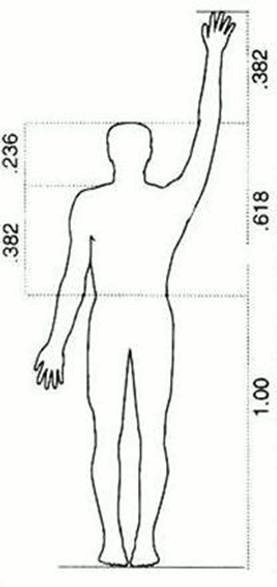# Trading Forex with Fibonacci (Part 1)

11/18/2015 9:00 am EST

Focus: FOREX

Huzefa Hamid

Senior Analyst, DailyForex.com

Huzefa Hamid, co-founder of the The Forex Room, gives an introduction to Fibonacci ratios and how to trade with them in part one of this three-part article series.

I am very pleased to have been invited by DailyForex.com and MoneyShow.com to write this short course on Fibonacci. Together, we’ll begin with the basics of Fibonacci and build on the concepts until we reach the stage of being able to plan trades. We’ll round off the course by looking at actual trade examples, including some trades that I have taken myself.

These concepts will be applied specifically to the currency markets, but they work in any market. Anything that has price, supply, and demand data can be analyzed and traded using Fibonacci ratios.

Part 1: The Fibonacci Numbers
So this is where it all begins: the “Fib numbers.” Leonardo Fibonacci was a 13th century Italian mathematician who made popular a simple sequence of numbers that came to be known as the “Fibonacci Number Sequence”.

The sequence is this: starting with 0 and 1, each number is the sum of the previous two numbers. So after 0 and 1, the next number is 1, followed by 2, followed by 3, then 5... you get the idea. The number sequence goes on forever, expanding to infinity:

0
1
1
2
3
5
8
13
21
34
55
89
144
233
377
610
987…

These numbers have some unique properties. Let’s take two consecutive numbers in the sequence: 21 and 34. If you divide one by the other, 21/34, you get 0.618. If you take any other two consecutive numbers, for example, 144 and 233, and divide one by the other, 144/233, again, you get 0.618. It doesn’t matter how far down the sequence you go, you will always arrive at 0.618 when you divide one number in the sequence by the next one along.

This particular ratio, 0.618 (or 61.8%) is often referred to as “The Golden Ratio” because so many traders find it to be a strong area of support or resistance on a chart.

Aside from 61.8%, there are other ratios present in the Fibonacci sequence. The next ratio is found by taking a Fibonacci number and dividing it by the number two places along in the sequence. For example, if we pick 21, we would divide it by 55, which is two places along. This gives 0.382 (or 38.2%). You would get 0.382 no matter which number you started with as long as you divide it by the number two places along. So, 89 divided by 233 is again 0.382.

Continuing with this idea, if you divide a Fibonacci number by a number three places along in the sequence, for example, 55 divided by 233, you would get a new ratio: 0.236, or 23.6%.

So far, we have discovered three common ratios in the Fib number sequence:

1. 0.236, or 23.6%
2. 0.382, or 38.2%
3. 0.618, or 61.8%, also known as “The Golden Ratio”

This is all great; it’s an interesting idea, but where does it lead us in our journey as traders?

Article Continues on Page 2…

|pagebreak|

The reason this series of numbers and its associated ratios are still being discussed centuries after first being widely known is because they are found everywhere in nature, and today, they are found in the markets.

For example, the human body is built around these ratios:From the foot to the navel, to the head, the common ratios of 0.236, 0.382, and 0.618 are found in the proportions of the human body.

The proportions of DNA strands are also in line with the Fibonacci ratios. So are the proportions of the moon to the Earth and even Saturn’s rings. The Greeks, over two thousand years ago, used the Golden Ratio when designing the proportions of the Parthenon, as did the Egyptians when calculating the size and height to build the Pyramids. Flowers more often than not have precise “Fib” numbers of petals, such as varieties of daisies with 55 petals and 89 petals.

Given that Fibonacci ratios are present from the smallest in DNA to the largest in planetary systems, it’s no surprise that these same ratios are seen in the way price moves in the market.

Let’s take a look at an example of price moving in harmony with The Golden Ratio. This is a daily chart of EUR/USD.

The price moves from the major low at point 1 to the major high at point 2, then retraces 61.8% of that distance before moving off again to continue the original upward trend.

What Ratios to Use When Trading
Aside from the three ratios discussed, there are other ratios that are used by traders (and also found in nature, for that matter). Three more common ratios are as follows:

1. 0.786: Square root of The Golden Ratio
2. 0.886: Square root of 0.786
3. 1.618: Inverse of The Golden Ratio, i.e. 1 divided by 0.618

To summarize:

1. Fibonacci begins with a simple sequence of numbers, each number being the sum of the previous two
2. Dividing consecutive numbers in the sequence, and numbers separated by one or two places, gives the common Fibonacci ratios: 0.236, 0.382 and 0.618. The last ratio, 61.8%, is known as “The Golden Ratio”
3. These ratios are found in nature and are also found in the way price moves in a market

Tomorrow in Part 2, we’ll look at Fibonacci ratios and how drawing retracements of those ratios on a chart will help you find levels for profit targets and stop losses on any trade.

By Huzefa Hamid, co-founder, The Forex Room

The Forex Room is a service that calls live trades to capture dozen of pips daily with low drawdown and educates its members on the set-ups.